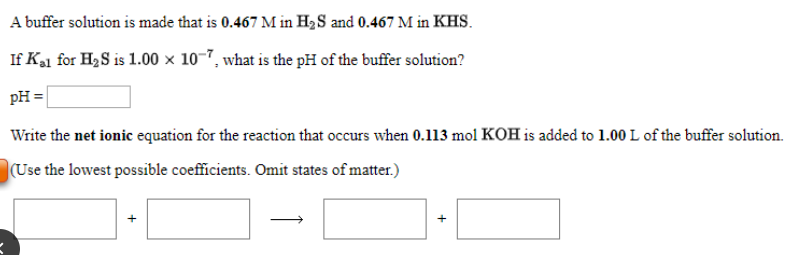# A buffer solution is made that is 0.467 M in H2S and 0.467 M in KHS. If Ka1 for H2S is 1.00 x 10^-7, what is the pH of the buffer solution? Write the net ionic equation for the reaction that occurs when 0.113 mol KOH is added to 1.00 L of the buffer solution. {Use the lowest possible coefficients. Omit states of matter.)